# ISEE Lower Level Math : How to add

## Example Questions

### Example Question #16 : Money And Time

George buys four items costing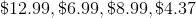Approximately how much does he pay in total?

Between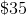and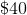Less than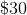Between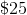andBetweenandMore thanBetweenandExplanation:

First, add up all of the values: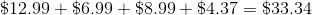Then, decide which of the answer choices approximates this value. Our answer is betweenand.

Another way to solve this problem is to round the four values to their nearest whole numbers and then sum those rounded values: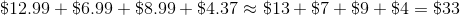### Example Question #11 : How To Add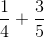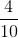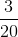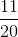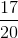Explanation:

First, find a common denominator. For these two fractions, it is 20. Then, change each fraction to reflect that new denominator.becomes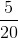.becomes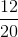. The next step is to add the numerators, which is 17. Remember, when adding fractions, we only add the numerators. Thus, your answer is### Example Question #12 : How To Add

Estimate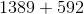.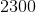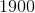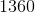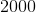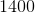Explanation:

To estimate, you must first round the numbers given. Round 1389 to 1400. Then, round 592 to 600. Combine 1400 and 600 to get 2000.

### Example Question #13 : How To Add

What is the standard form for writing five hundred twenty-two thousand twenty?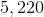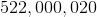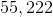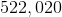None of theseExplanation:

To determine the standard form for five hundred twenty-two thousand twenty, it is best to take it step by step:

• five hundred thousand =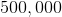• twenty-two thousand =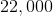• twenty =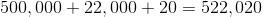### Example Question #14 : How To Add

Which of the following is true about the set below?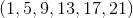The numbers are all odd

The numbers are all prime

The numbers are all divisble by 4

None of these

The numbers are all multiples of 4

The numbers are all odd

Explanation:The numbers in the set are all odd, meaning that they are not divisble by 2.

While the numbers in the set increase by 4 sequentially, they are not divisible by 4, and therefore are not multiples of 4.

Thus, the best answer is that they are all odd.

Additionally, the numbers are not prime because 9 and 21 are both divisible by 3.

### Example Question #15 : How To Add

Jill is getting ready to go to school. She wakes up an hour before class starts and it takes her 15 minutes to walk to school. If class starts at 8:00, which of the following best describes when she must leave home to be at class on time?

She must leave in 45 minutes in order to leave at 7:45.

She must leave in 45 minutes in order to leave at 7:25.

She must leave in 35 minutes in order to leave at 7:45.

She must leave in 40 minutes in order to leave at 7:45.

She must leave in 45 minutes in order to leave at 7:45.

Explanation:

Given that Jill's class starts at 8:00 and that it takes her 15 minutes to walk there, she must leave at 7:45. Since she woke up an hour before class starts, at 7 am, this means that she must leave in 45 minutes. Therefore, the correct answer is:

She must leave in 45 minutes in order to leave at 7:45.

### Example Question #16 : How To AddExplanation: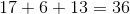### Example Question #17 : How To Add

What is the value ofin the equation?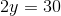Explanation:To solve, you will need to getalone on the left side of the equation. Do this by dividing both sides by 2.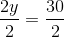The left side cancels.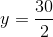Reduce the right side.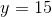### Example Question #18 : How To Add

Simplify: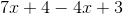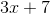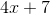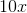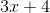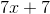Explanation:

To simplify, you must rearrange the expression to group like-terms together.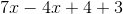Like-terms can be simplified.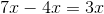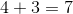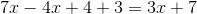This results in, which is the correct answer.

### Example Question #19 : How To Add

Lisa has been tutoring students every day for the past six days. The first three days, she tutored 5 students each day. The last three days she tutored 3 students each day. On average, how many students did she tutor each day?Explanation:

The average consists of the sum of the number of students tutored for the  6 days, divided by 6. Thus, the average would be calculated with this equation: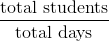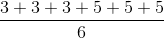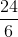This is equal to.

### All ISEE Lower Level Math Resources